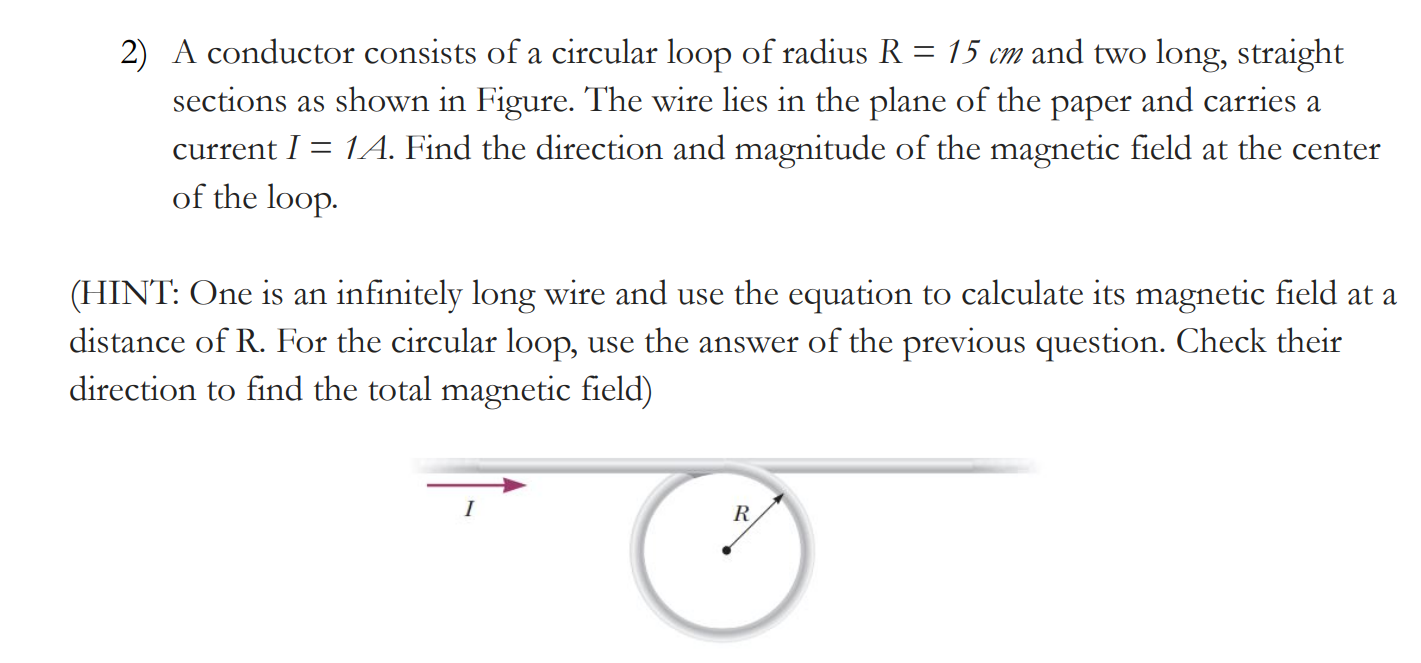Home / Expert Answers / Physics / i-39-m-stuck-on-this-physics-problem-and-really-need-some-help-understanding-it-physics-isn-39-t-exa-pa852

# (Solved): I'm stuck on this physics problem and really need some help understanding it.Physics isn't exactly m ...

I'm stuck on this physics problem and really need some help understanding it.

Physics isn't exactly my strong suit, so I'd appreciate a breakdown of the answer, like step-by-step, so I can get what's going on.2) A conductor consists of a circular loop of radius and two long, straight sections as shown in Figure. The wire lies in the plane of the paper and carries a current . Find the direction and magnitude of the magnetic field at the center of the loop. (HINT: One is an infinitely long wire and use the equation to calculate its magnetic field at a distance of R. For the circular loop, use the answer of the previous question. Check their direction to find the total magnetic field)

We have an Answer from Expert